# Antibiotics utilization in Denmark: using R to solve practical tasks in epidemiology

## Intro

This is a blog post-chaperon for the R-Ladies meet-up Abuja. We are talking R in epidemiology, practical aspects of being R-user and going through data-supported example of how to use R for data aggregation and visualization.

## Getting started

library(tidyverse)
library(magrittr)
library(wesanderson)


link_list_dk <- list(
)

# ATC codes are stored in files
seq_atc <- c(1:24)

# drugs names
eng_drug <- read_delim(link_list_dk[["atc_groups.txt"]], delim = ";", col_names = c(paste0("V", c(1:6))), col_types = cols(V1 = col_character(), V2 = col_character(), V3 = col_character(), V4 = col_character(), V5 = col_character(), V6 = col_character())) %>%
# keep drug classes labels in English
filter(V5 == "1") %>%
select(ATC = V1,
drug = V2,
unit_dk = V4)

# drugs data
atc_data <- map(atc_code_data_list, ~read_delim(file = .x, delim = ";", trim_ws = T, col_names = c(paste0("V", c(1:14))), col_types = cols(V1 = col_character(), V2 = col_character(), V3 = col_character(), V4 = col_character(), V5 = col_character(),V6 = col_character(), V7 = col_character(), V8 = col_character(), V9 = col_character(), V10 = col_character(), V11 = col_character(), V12 = col_character(), V13 = col_character(), V14 = col_character()))) %>%
# bind atc_data from all years
bind_rows()

# population data
pop_data <- read_delim(link_list_dk[["population_data.txt"]], delim = ";", col_names = c(paste0("V", c(1:7))), col_types = cols(V1 = col_character(), V2 = col_character(), V3 = col_character(), V4 = col_character(), V5 = col_character(), V6 = col_character(), V7 = col_character())) %>%
# rename and keep columns
select(year = V1,
region_text = V2,
region = V3,
gender = V4,
age = V5,
denominator_per_year = V6) %>%
# human-reading friendly label on sex categories
mutate(
gender_text = case_when(
gender == "1" ~ "men",
gender == "2" ~ "women",
T ~ as.character(gender)
)
) %>%
# make numeric variables
mutate_at(vars(year, age, denominator_per_year), as.numeric) %>%
arrange(year, age, region, gender)


## Explore data structure

atc_data %>% skimr::skim()

Name Piped data
Number of rows 28814496
Number of columns 14
_______________________
Column type frequency:
character 14
________________________
Group variables None

Table 1: Data summary

Variable type: character

skim_variable n_missing complete_rate min max empty n_unique whitespace
V1 0 1.00 1 7 0 2967 0
V2 0 1.00 4 4 0 24 0
V3 0 1.00 1 1 0 3 0
V4 0 1.00 1 1 0 6 0
V5 0 1.00 1 1 0 4 0
V6 0 1.00 1 5 0 104 0
V7 513229 0.98 1 7 0 62562 0
V8 513229 0.98 1 7 0 86412 0
V9 186071 0.99 1 8 0 79983 0
V10 637631 0.98 1 7 0 58064 0
V11 1309019 0.95 1 6 0 33861 0
V12 1309167 0.95 1 6 0 8403 0
V13 368860 0.99 1 3 0 101 0
V14 28814496 0.00 NA NA 0 0 0

## Clean data

atc_data %<>%
# rename and keep columns
rename(
ATC = V1,
year = V2,
sector = V3,
region = V4,
gender = V5, # 0 - both; 1 - male; 2 - female; A - age in categories
age = V6,
number_of_people = V7,
patients_per_1000_inhabitants = V8,
turnover = V9,
regional_grant_paid = V10,
quantity_sold_1000_units = V11,
quantity_sold_units_per_unit_1000_inhabitants_per_day = V12,
percentage_of_sales_in_the_primary_sector = V13
)

atc_data %<>%
# clean columns names and set-up labels
mutate(
year = as.numeric(year),
region_text = case_when(
region == "0" ~ "DK",
region == "1" ~ "Region Hovedstaden",
region == "2" ~ "Region Midtjylland",
region == "3" ~ "Region Nordjylland",
region == "4" ~ "Region Sjælland",
region == "5" ~ "Region Syddanmark",
T ~ NA_character_
),
gender_text = case_when(
gender == "0" ~ "both sexes",
gender == "1" ~ "men",
gender == "2" ~ "women",
T ~ as.character(gender)
)
) %>%
mutate_at(vars(turnover, regional_grant_paid, quantity_sold_1000_units, quantity_sold_units_per_unit_1000_inhabitants_per_day, number_of_people, patients_per_1000_inhabitants), as.numeric) %>%
select(-V14) %>%
filter(
region == "0",
sector == "0",
gender != "0"
)

atc_data %<>%
# deal with non-numeric age in groups
filter(
str_detect(age, "[0-9][0-9][0-9]")
) %>%
mutate(
age = parse_number(age)
) %>%
select(year, ATC, gender, age, number_of_people, patients_per_1000_inhabitants, region_text, gender_text)

atc_data %>% slice(1:100)

## # A tibble: 100 × 8
##     year ATC   gender   age number_of_people patients_per_1000_inha… region_text
##    <dbl> <chr> <chr>  <dbl>            <dbl>                   <dbl> <chr>
##  1  1999 A     2          0             6824                   212.  DK
##  2  1999 A     1          0             7760                   228.  DK
##  3  1999 A     2          1             2633                    79.6 DK
##  4  1999 A     1          1             2872                    82.3 DK
##  5  1999 A     2          2             1049                    31.6 DK
##  6  1999 A     1          2             1132                    32.3 DK
##  7  1999 A     2          3              545                    15.8 DK
##  8  1999 A     1          3              597                    16.4 DK
##  9  1999 A     2          4              412                    11.8 DK
## 10  1999 A     1          4              482                    13.2 DK
## # … with 90 more rows, and 1 more variable: gender_text <chr>


We will examine the utilization of antimicrobials for systemic use by sex and age group and assess temporal trends. For this, we, first, need to specify the groups of codes we are interested in.

## Antimictbials for systemic use

regex_AB <- "^J01$" regex_TETRACYCLINES <- "^J01A$"
regex_AMPHENICOLS <- "^J01B$" regex_BETALACTAMS <- "^J01C$"
regex_OTHER_BETALACTAMS <- "^J01D$" regex_SULFONAMIDES_TRIMETHOPRIM <- "^J01E$"
regex_MACROLIDES_LINCOSAMIDES_STREPTOGRAMINS <- "^J01F$" regex_AMINOGLYCOSIDES <- "^J01G$"
regex_QUINOLONES <- "^J01M$" # regex_COMB <- "^J01R$"
# regex_OTHER <- "^J01X\$"

# combine all ATC codes in one regex string
all_regex <- paste(regex_AB, regex_AB, regex_TETRACYCLINES, regex_AMPHENICOLS, regex_BETALACTAMS, regex_OTHER_BETALACTAMS, regex_SULFONAMIDES_TRIMETHOPRIM, regex_MACROLIDES_LINCOSAMIDES_STREPTOGRAMINS, regex_AMINOGLYCOSIDES, regex_QUINOLONES, sep = "|")


## Keep only the ATC codes we are interested in

atc_data %<>% filter(str_detect(ATC, all_regex))


## Merge/join with the drugs names dataset

atc_data %<>% left_join(eng_drug)

## Joining, by = "ATC"


## Merge/join with the population data

# join with the population data
atc_data %<>% left_join(pop_data)

## Joining, by = c("year", "gender", "age", "region_text", "gender_text")

# create age bands
atc_data %<>% mutate(
age_cat = cut(age, c(-Inf, 18, 25, 30, 35, 40, 45, 50, 55, 60, 65, 70, Inf))
)

atc_data %>% count(age_cat)

## # A tibble: 12 × 2
##    age_cat       n
##    <fct>     <int>
##  1 (-Inf,18]  5873
##  2 (18,25]    2280
##  3 (25,30]    1613
##  4 (30,35]    1601
##  5 (35,40]    1607
##  6 (40,45]    1583
##  7 (45,50]    1552
##  8 (50,55]    1533
##  9 (55,60]    1534
## 10 (60,65]    1537
## 11 (65,70]    1511
## 12 (70, Inf]  7155

atc_data %>%
filter(str_detect(ATC, regex_AB)) %>%
ggplot(aes(x = year, y = patients_per_1000_inhabitants, color = gender_text)) +
geom_point() +
theme_light(base_size = 14) +
scale_x_continuous(breaks = c(seq(1999, 2019, 10))) +
scale_color_manual(values = wes_palette(name = "GrandBudapest1", type = "discrete")) +
theme(plot.caption = element_text(hjust = 0, size = 10),
legend.position = "bottom",
panel.spacing = unit(0.8, "cm")) +
guides(colour = guide_legend("Sex", ncol = 2)) +
labs(y = "Patients\nper 1,000 population", title = "Antimicrobials for systemic use in Denmark by sex", subtitle = "1996-2019", caption = "Elena Dudukina | @evpatora | medstat.dk")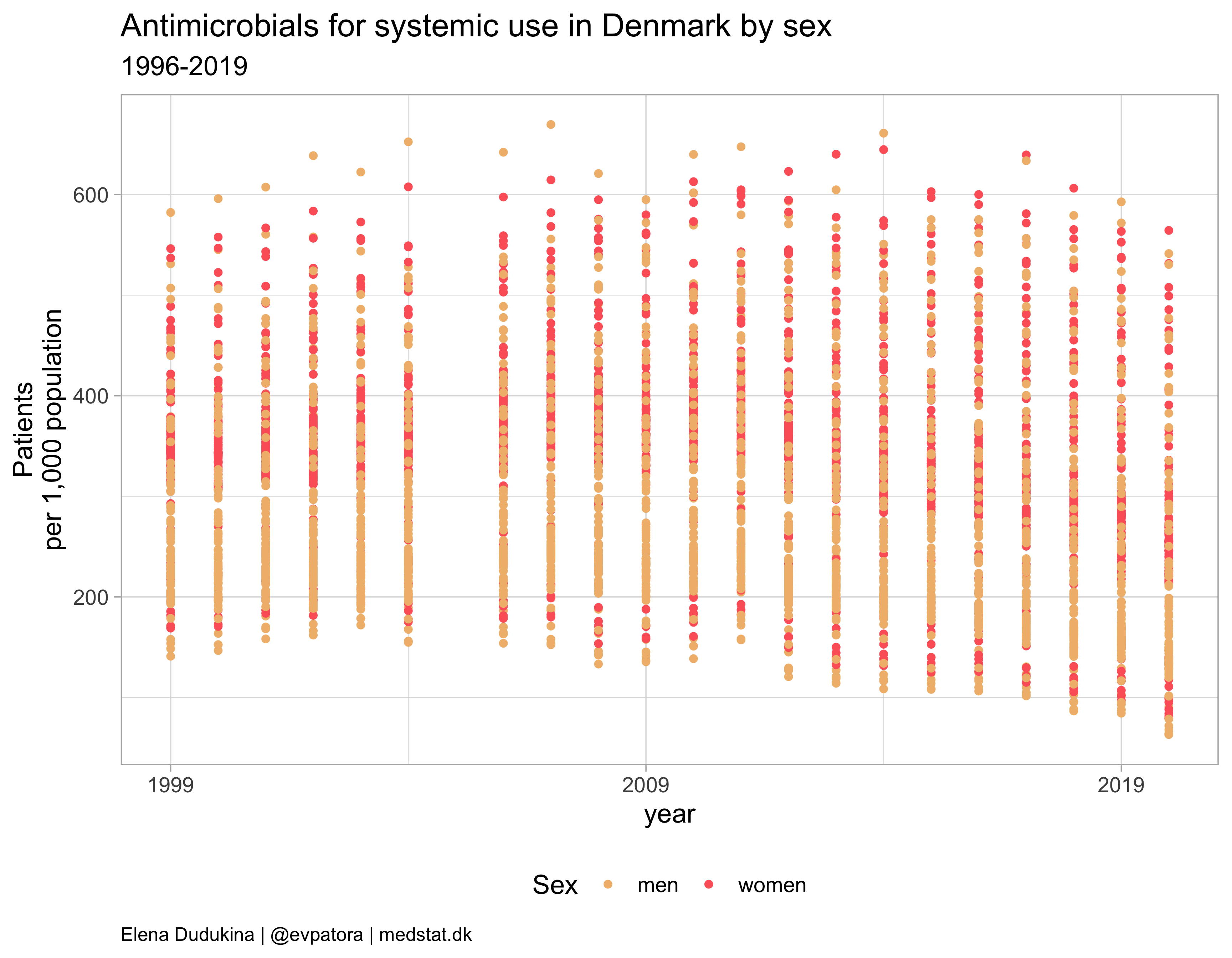## What is the problem with the data?

The data is for yearly drug use per age category in 1-year bands, while we need the drug use per wider age category.

# create drug use rates for age categories
atc_data %<>%
group_by(gender, age_cat, ATC, year) %>%
mutate(
numerator = sum(number_of_people),
denominator = sum(denominator_per_year),
patients_per_1000_inhabitants = numerator / denominator * 1e3
) %>%
ungroup() %>%
distinct(gender, age_cat, ATC, year, patients_per_1000_inhabitants, .keep_all = T) %>%
select(-age)


## Antibiotics use by year, sex, and age group

atc_data %>%
filter(str_detect(ATC, regex_AB)) %>%
ggplot(aes(x = year, y = patients_per_1000_inhabitants, color = age_cat)) +
geom_point() +
facet_grid(cols = vars(gender_text)) +
theme_light(base_size = 14) +
scale_x_continuous(breaks = c(seq(1999, 2019, 10))) +
scale_color_manual(values = wes_palette(name = "GrandBudapest1", type = "continuous", n = 12)) +
theme(plot.caption = element_text(hjust = 0, size = 10),
legend.position = "bottom",
panel.spacing = unit(0.8, "cm")) +
guides(color = guide_legend("Age, categories", ncol = 4)) +
labs(y = "Patients\nper 1,000 population", title = "Antimicrobials for systemic use in Denmark by sex", subtitle = "1999-2020", caption = "Elena Dudukina | @evpatora | medstat.dk")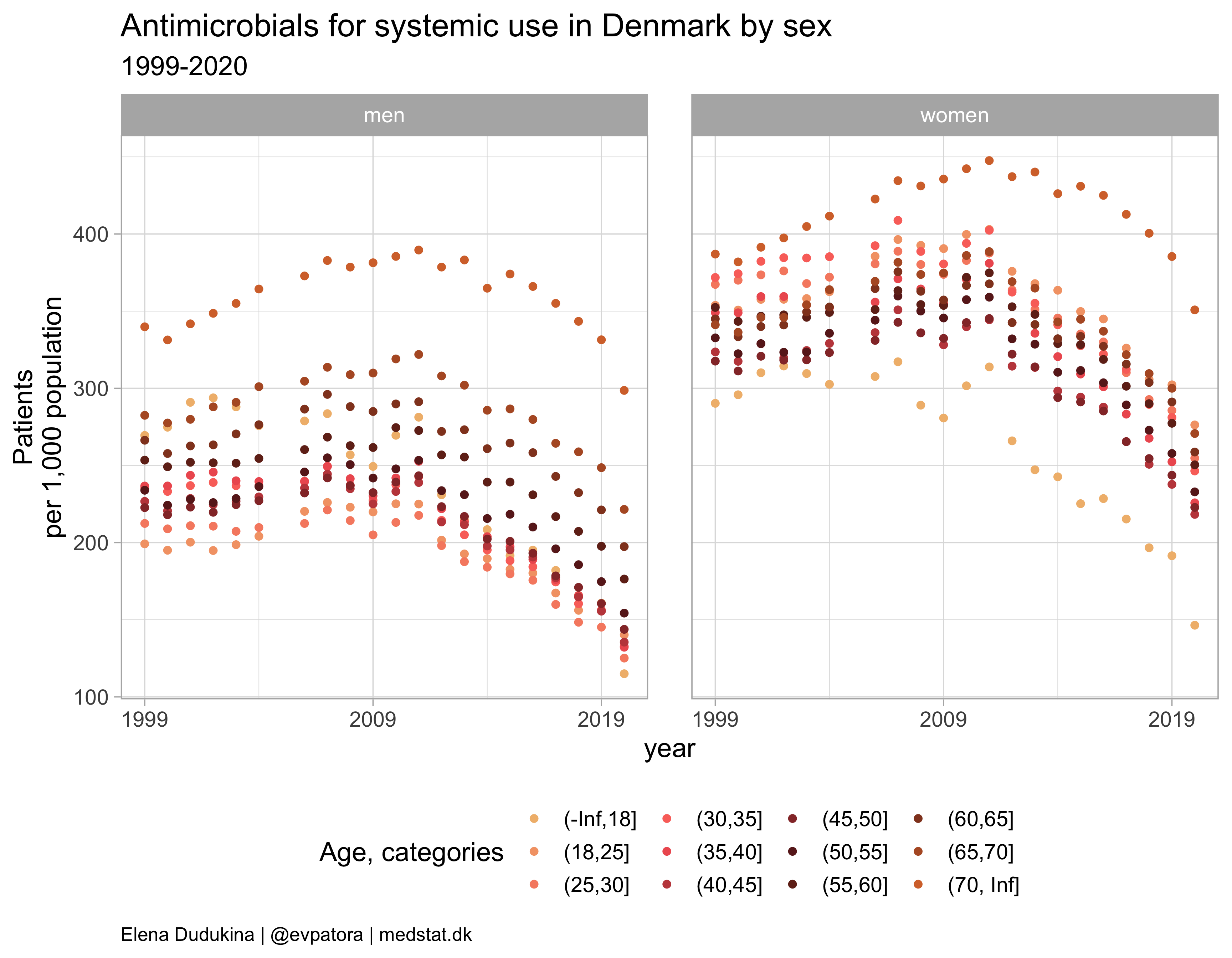atc_data %>%
filter(str_detect(ATC, regex_AB)) %>%
mutate(label = if_else(year == max(year), as.character(age_cat), NA_character_)) %>%
ggplot(aes(x = year, y = patients_per_1000_inhabitants, color = age_cat)) +
geom_path(position = position_dodge(width = 1)) +
facet_grid(cols = vars(gender_text)) +
theme_light(base_size = 14) +
scale_x_continuous(limits = c(1999, 2026), breaks = c(seq(1999, 2020, 10))) +
scale_y_continuous(position = "right") +
scale_color_manual(values = wes_palette(name = "GrandBudapest1", type = "continuous", n = 12)) +
theme(plot.caption = element_text(hjust = 0, size = 10),
legend.position = "none",
panel.spacing = unit(0.8, "cm")) +
labs(y = "Patients\nper 1,000 population\n", title = "Antimicrobials for systemic use in Denmark by sex", subtitle = "1999-2020", caption = "Elena Dudukina | @evpatora | medstat.dk") +
ggrepel::geom_label_repel(aes(label = label, na.rm = TRUE), direction = "y", segment.size = 0.2, segment.colour = "grey", show.legend = F, size = 3.5, nudge_x = 5, hjust = 0.5, max.overlaps = 20)

## Warning: Ignoring unknown aesthetics: na.rm

## Warning: Removed 6 row(s) containing missing values (geom_path).

## Warning: Removed 480 rows containing missing values (geom_label_repel).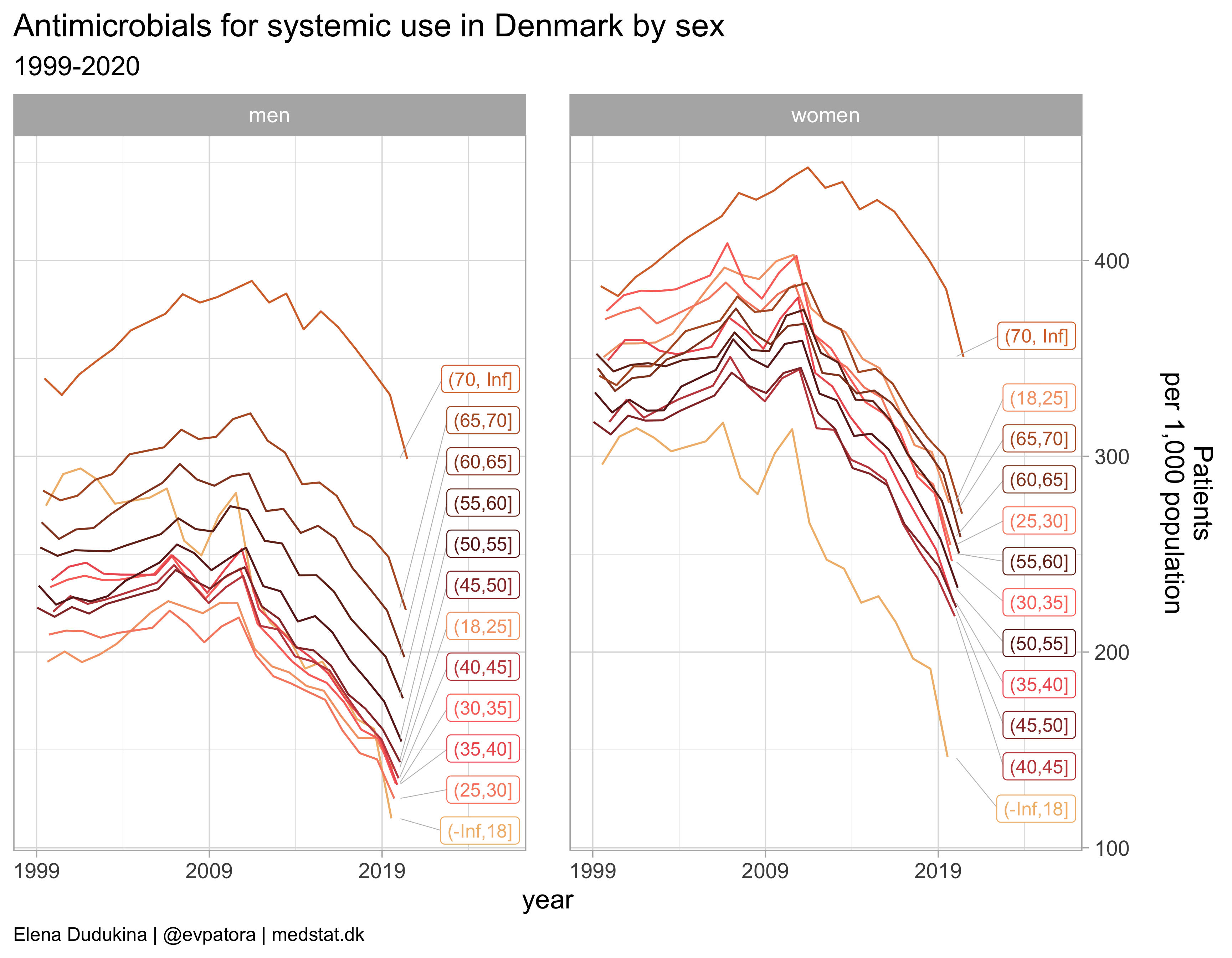## Focus on utilization rate instead of secular trend

atc_data %>%
filter(str_detect(ATC, regex_AB)) %>%
mutate(age_cat = fct_rev(age_cat)) %>%
group_by(age_cat, gender) %>%
mutate(label = case_when(
patients_per_1000_inhabitants == max(patients_per_1000_inhabitants) ~ paste0(round(median(patients_per_1000_inhabitants, 1)), " (", round(quantile(patients_per_1000_inhabitants, probs = 0.25), 1), "-",  round(quantile(patients_per_1000_inhabitants, probs = 0.75), 1), ")"),
T ~ NA_character_
)) %>%
ungroup() %>%
ggplot(aes(x = age_cat, y = patients_per_1000_inhabitants, color = year)) +
geom_point() +
geom_boxplot(alpha = 0.3) +
facet_grid(cols = vars(gender_text)) +
theme_light(base_size = 14) +
scale_color_gradientn(colours = RColorBrewer::brewer.pal(name = "Blues", n = 21)) +
coord_flip() +
theme(plot.caption = element_text(hjust = 0, size = 10),
legend.position = "bottom", legend.text = element_text(size = 7),
panel.spacing = unit(0.8, "cm")) +
labs(y = "Patients\nper 1,000 population\n", title = "Antimicrobials for systemic use in Denmark by sex", subtitle = "1999-2020", caption = "Elena Dudukina | @evpatora | medstat.dk", x = "Age, years") +
guides(color = guide_colorbar("Calendar year", barheight = 5))  +
ggrepel::geom_label_repel(aes(label = label, na.rm = TRUE), direction = "x", segment.size = 0.2, segment.colour = "grey", show.legend = F, size = 2, nudge_y = 1, hjust = 0.5, max.overlaps = 20)

## Warning in RColorBrewer::brewer.pal(name = "Blues", n = 21): n too large, allowed maximum for palette Blues is 9
## Returning the palette you asked for with that many colors

## Warning: Ignoring unknown aesthetics: na.rm

## Warning: Removed 480 rows containing missing values (geom_label_repel).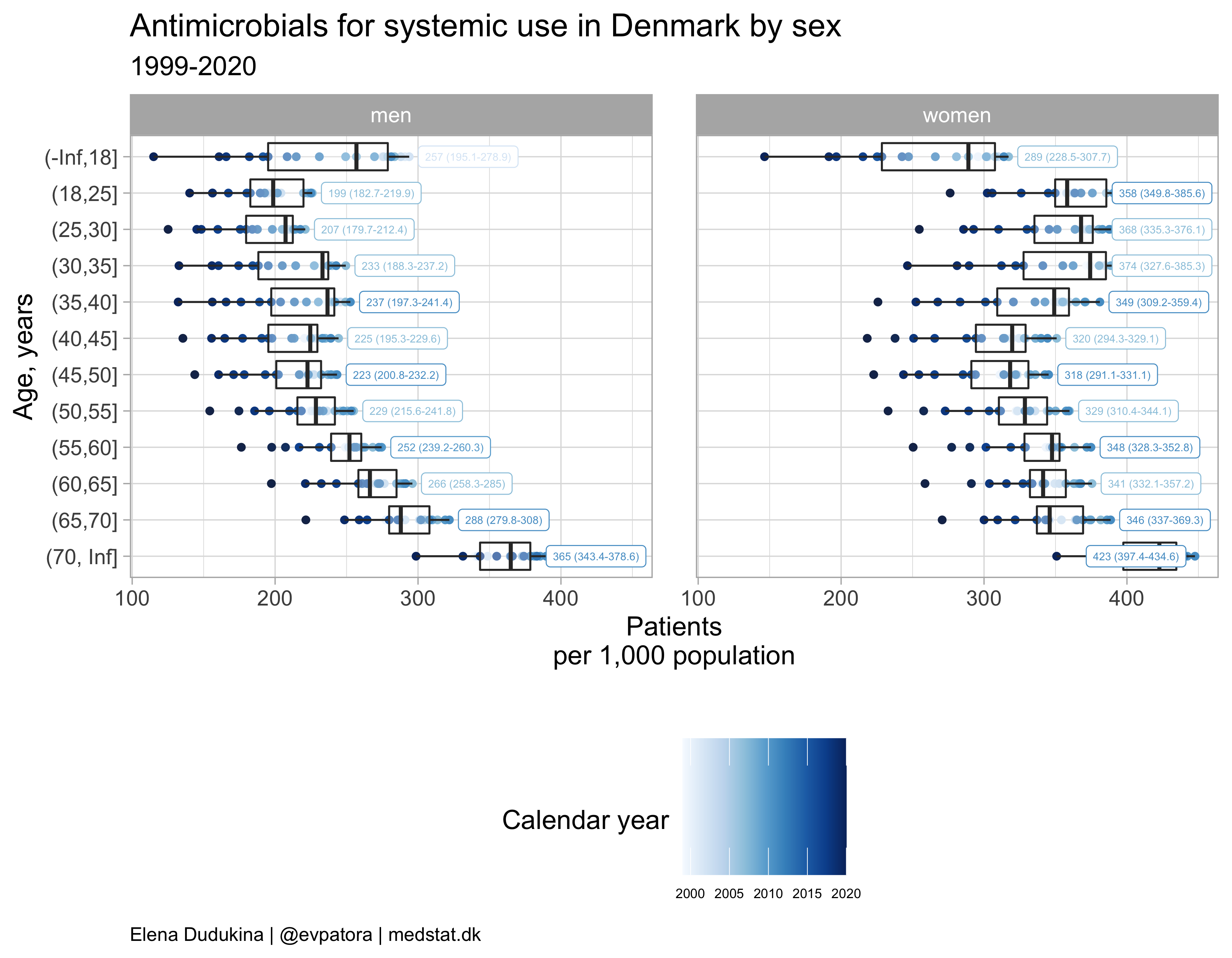## Output similar plots for each antimicrobial ATC sub-class

plot_use <- function(atc_data, drug_regex, title){
atc_data %>%
filter(str_detect(ATC, {{drug_regex}})) %>%
mutate(label = if_else(year == max(year), as.character(age_cat), NA_character_)) %>%
ggplot(aes(x = year, y = patients_per_1000_inhabitants, color = age_cat)) +
geom_path(position = position_dodge(width = 1)) +
facet_grid(cols = vars(gender_text)) +
theme_light(base_size = 14) +
scale_x_continuous(limits = c(1999, 2026), breaks = c(seq(1999, 2020, 10))) +
scale_y_continuous(position = "right") +
scale_color_manual(values = wes_palette(name = "GrandBudapest1", type = "continuous", n = 12)) +
theme(plot.caption = element_text(hjust = 0, size = 10),
legend.position = "none",
panel.spacing = unit(0.8, "cm")) +
labs(y = "Patients\nper 1,000 population\n", title = paste0(title, " use in Denmark by sex"), subtitle = "1999-2020", caption = "Elena Dudukina | @evpatora | medstat.dk") +
ggrepel::geom_label_repel(aes(label = label, na.rm = TRUE), direction = "y", segment.size = 0.2, segment.colour = "grey", show.legend = F, size = 3.5, nudge_x = 5, hjust = 0.5, max.overlaps = 20)
}

list_regex <- list(regex_TETRACYCLINES, regex_AMPHENICOLS, regex_BETALACTAMS, regex_OTHER_BETALACTAMS, regex_SULFONAMIDES_TRIMETHOPRIM, regex_MACROLIDES_LINCOSAMIDES_STREPTOGRAMINS, regex_AMINOGLYCOSIDES, regex_QUINOLONES)
list_title <- list("Tetracyclines", "Amphenicols", "Beta-lactam antibacterials, penicillins", "Other beta-lactams", "Sulfonamides or trimetoprim", "Macrolides, lincosamides, streptogramins", "Aminoglycosides", "Quinolones")
list_plots <- map2(list_regex, list_title, ~plot_use(atc_data = atc_data, drug_regex = .x, title = .y))


## Results

### Tetracyclines

list_plots[]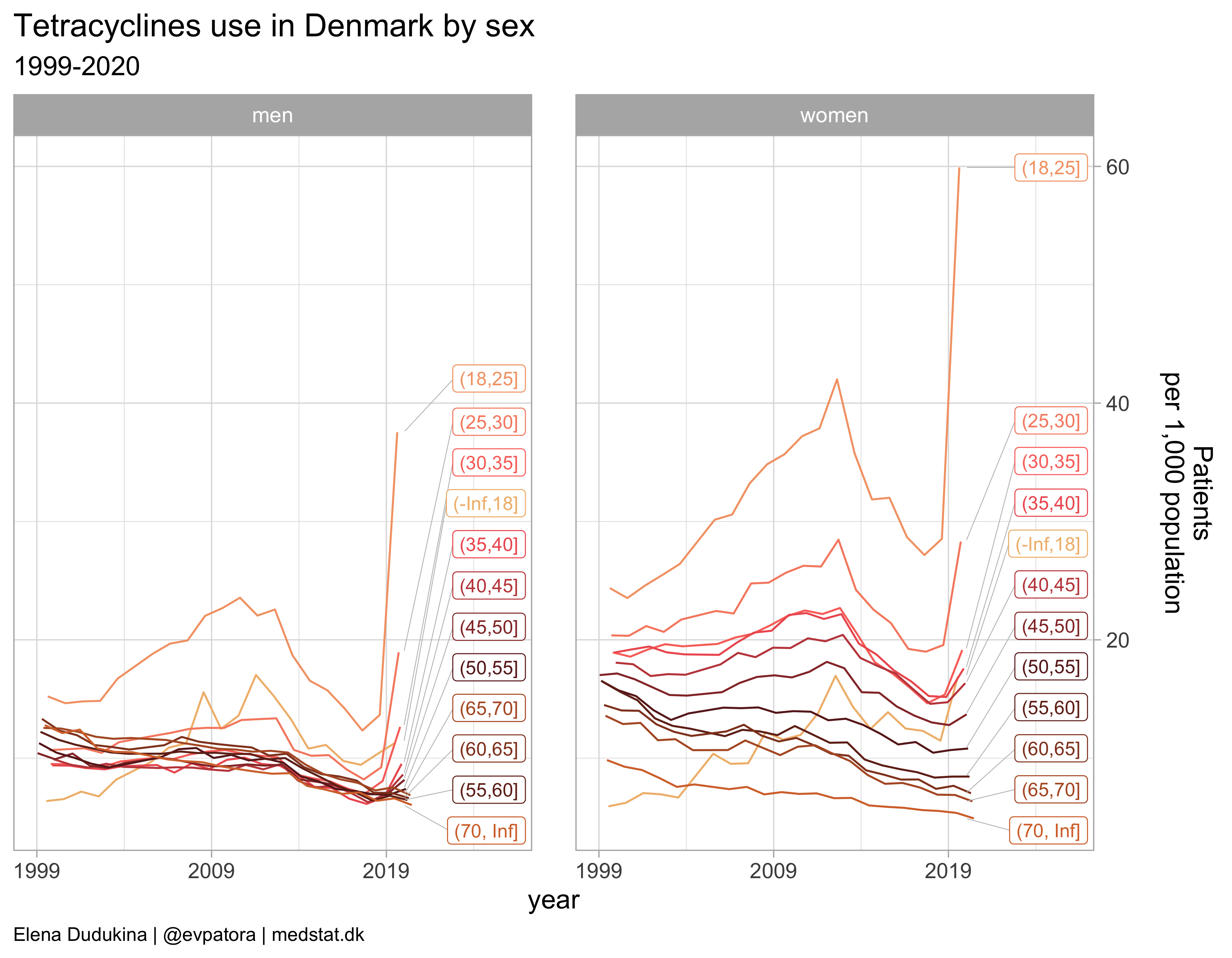### Amphenicols

list_plots[]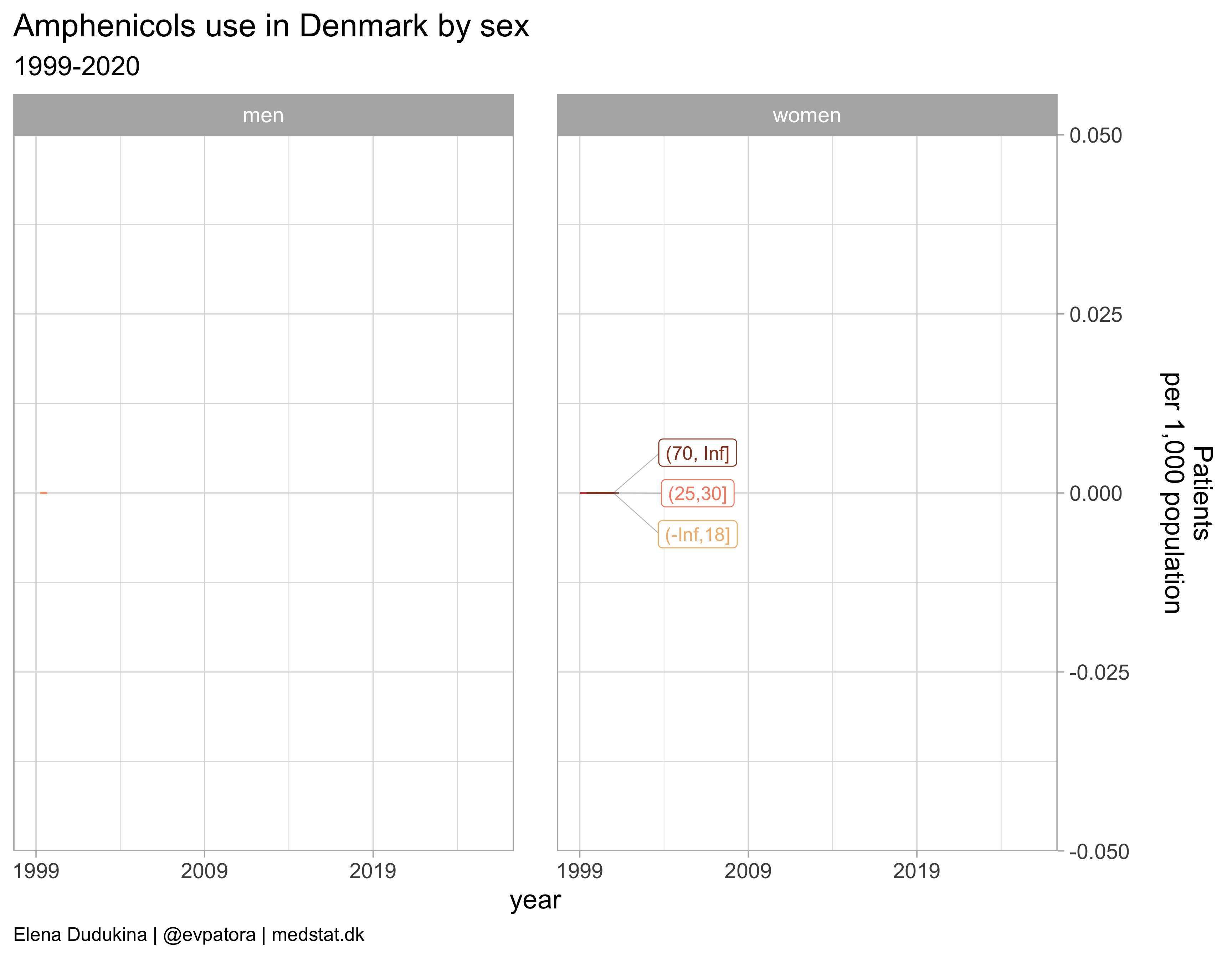### Beta-lactam antibacterials, penicillins

list_plots[]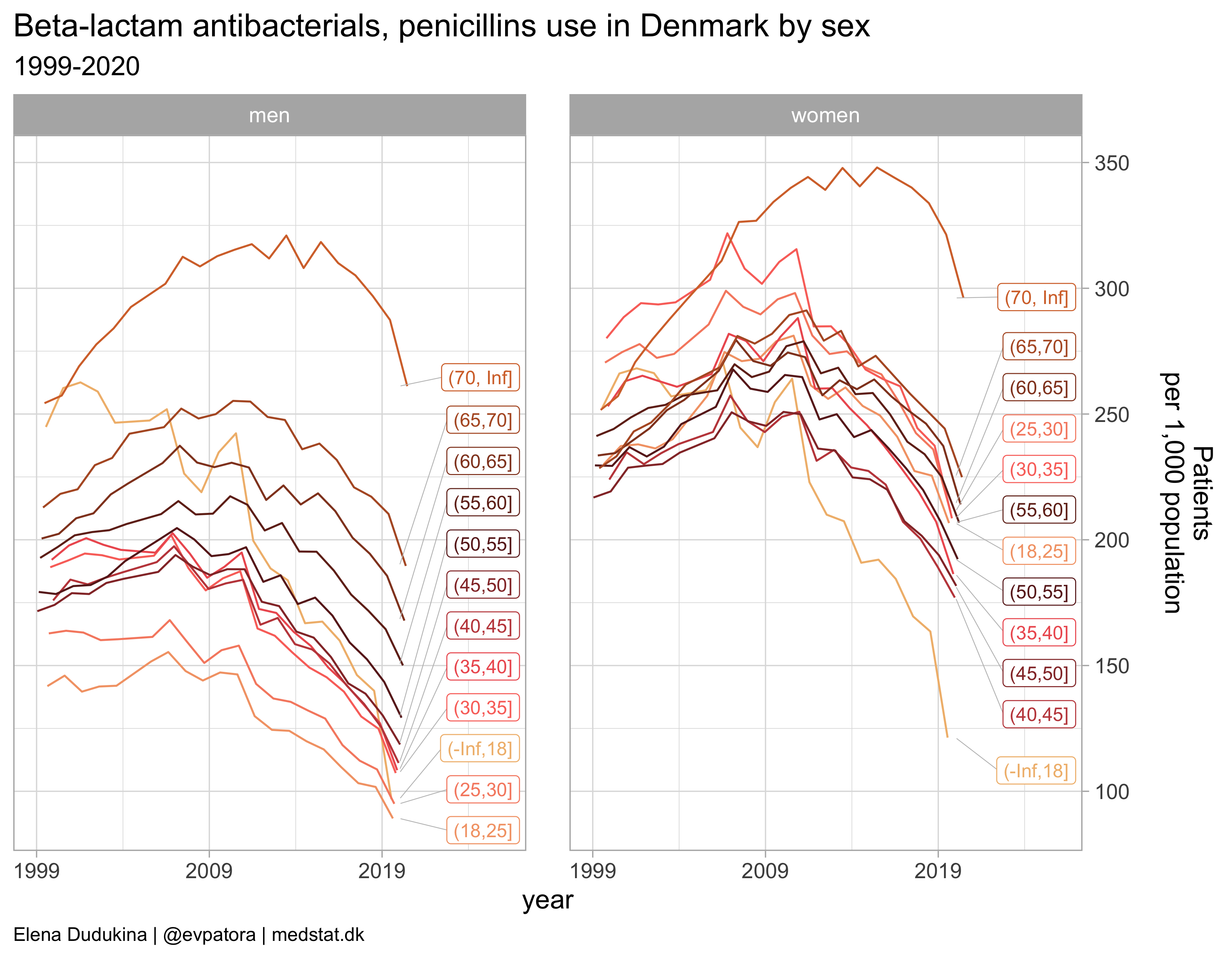### Other beta-lactams

list_plots[]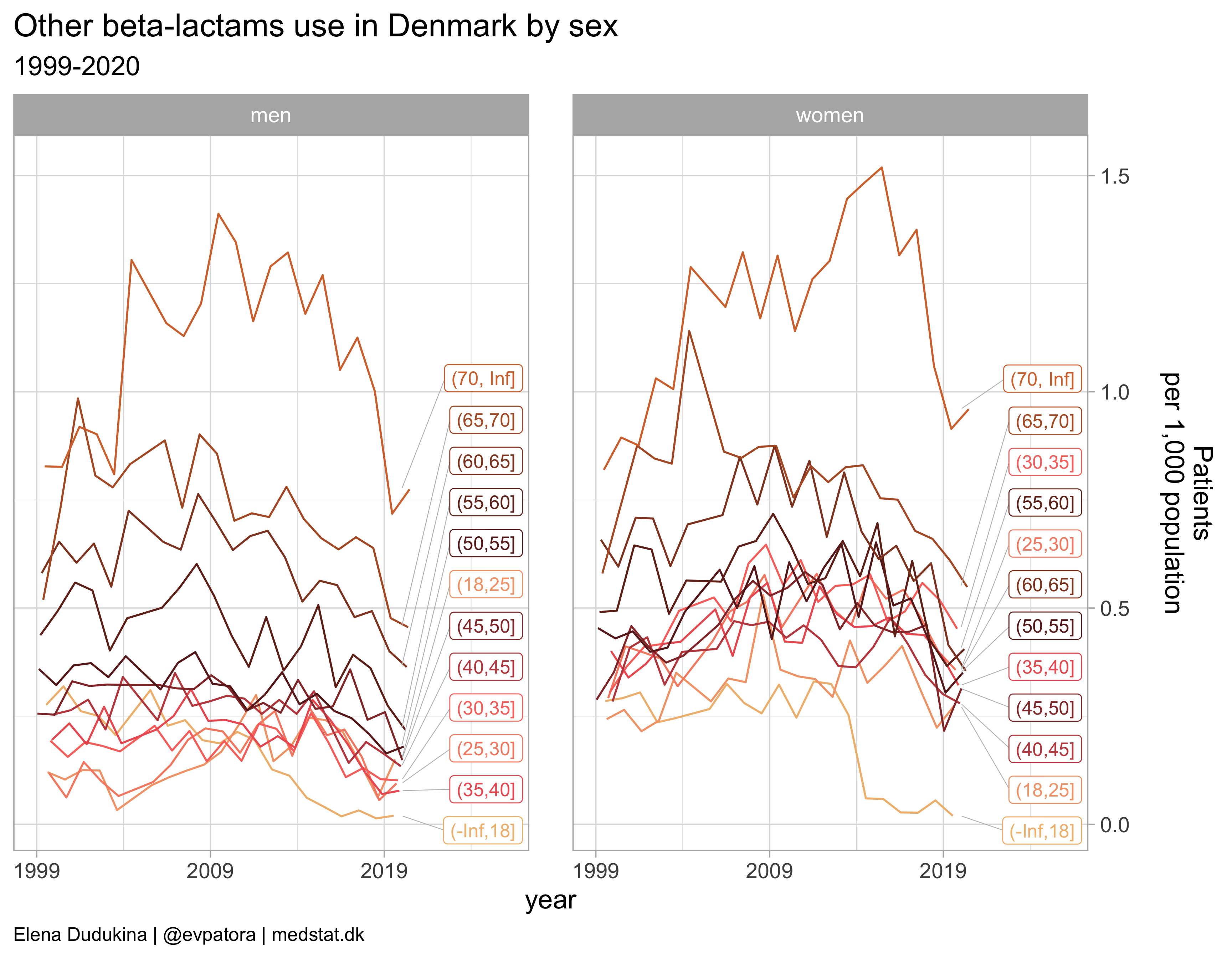### Sulfonamides or trimetoprim

list_plots[]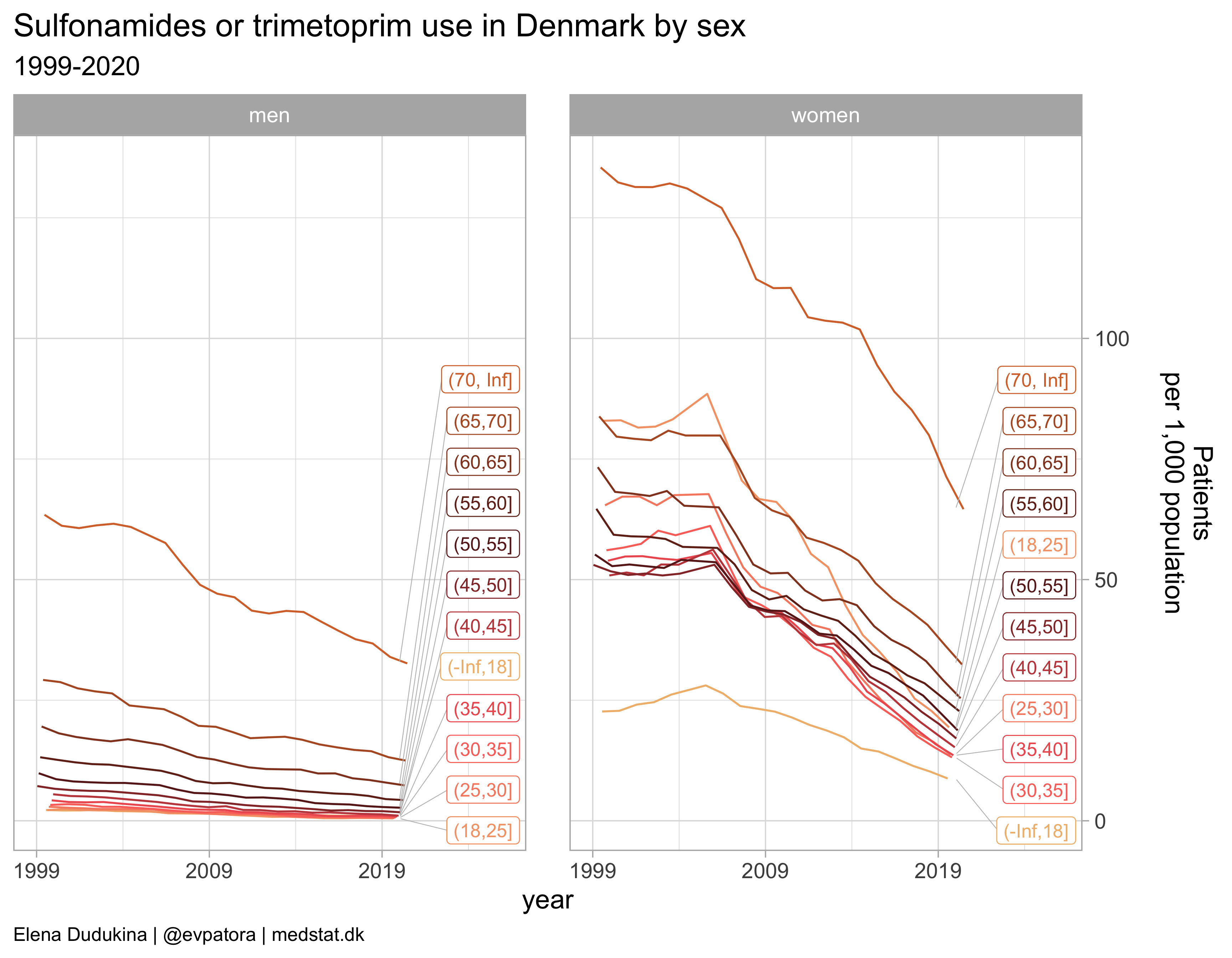### Macrolides, lincosamides, streptogramins

list_plots[]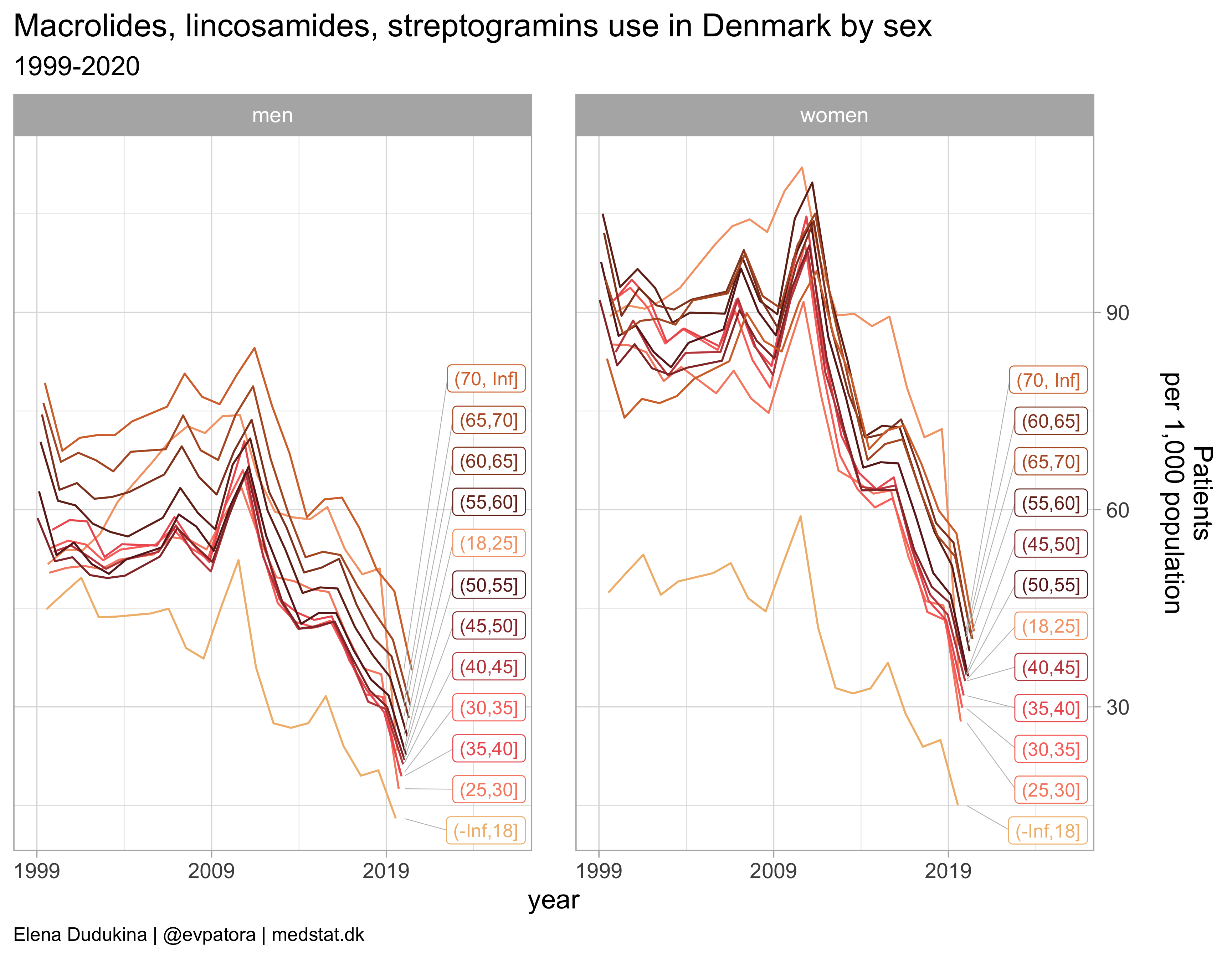### Aminoglycosides

list_plots[]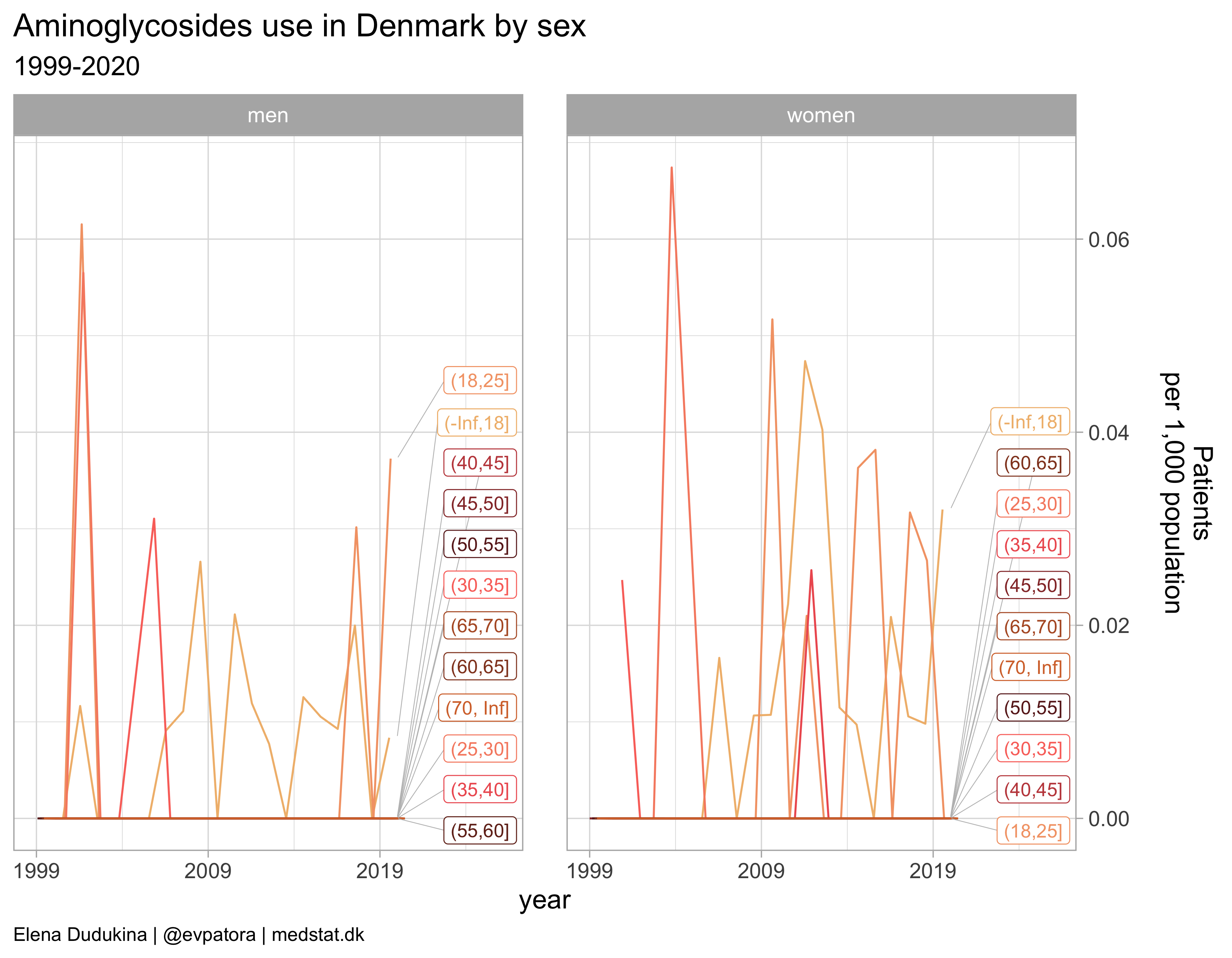### Quinolones

list_plots[]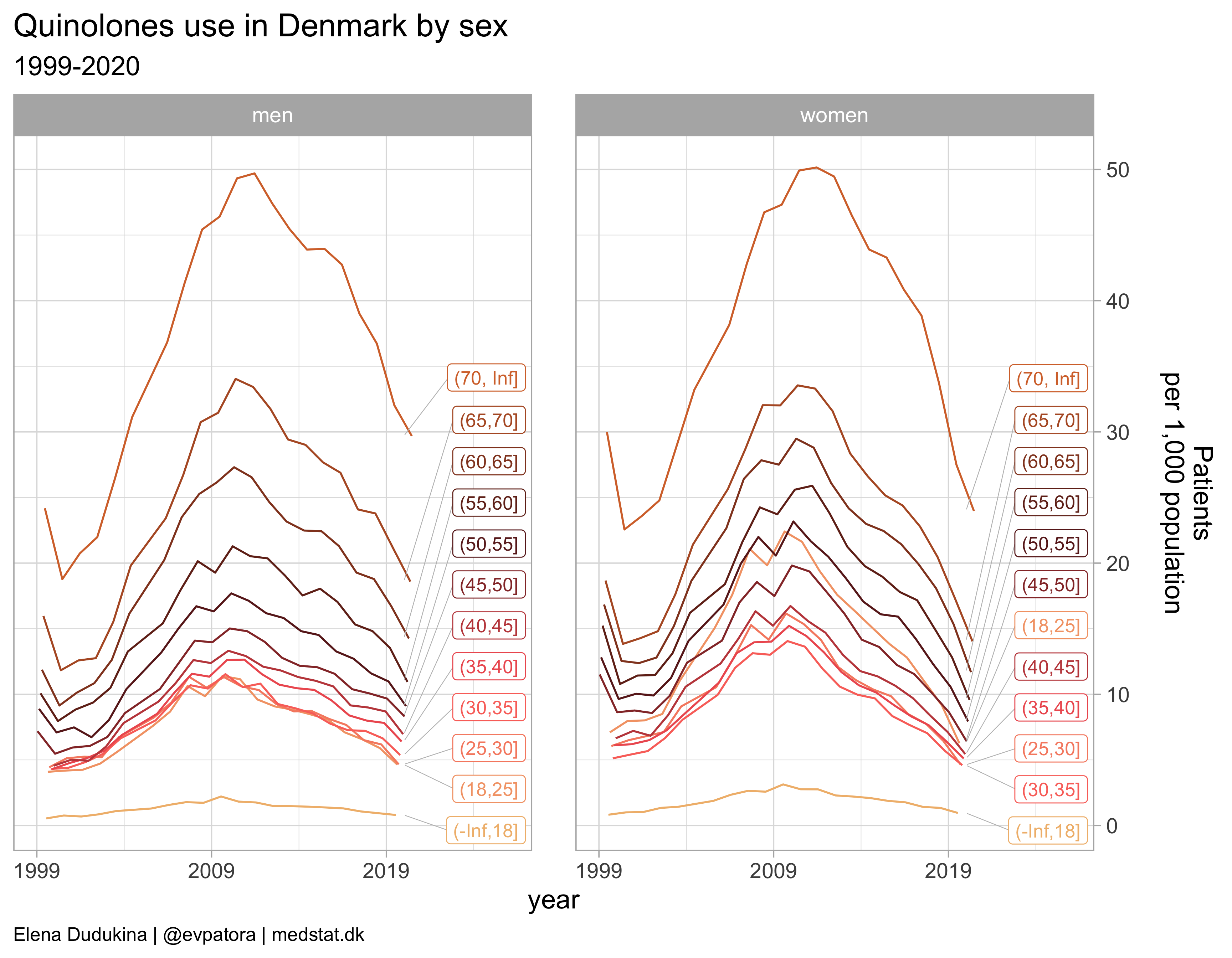### Final results table for the specific age groups of interest: children and elderly in 2019-2020

atc_data %>%
filter(age_cat %in% c("(-Inf,18]", "(65,70]", "(70, Inf]"), year %in% c(2019:2020)) %>%
mutate(patients_per_1000_inhabitants = round(patients_per_1000_inhabitants, 1)) %>%
select(Gender = gender_text, Age = age_cat, Year = year, ATC, Drug = drug, "Use, per 1000 capita" = patients_per_1000_inhabitants) %>%
pivot_wider(names_from = c(Age, Gender), values_from = "Use, per 1000 capita", names_sep = ", ") %>%
arrange(ATC) %>%
gt::gt(.) %>%
title = "Antibacterials for systemic use in vulnerable age groups by sex, Denmark, 2019-2020",
subtitle = "Drug use, per 1000 capita of the underlying population (age group, sex)"
)


Antibacterials for systemic use in vulnerable age groups by sex, Denmark, 2019-2020
Drug use, per 1000 capita of the underlying population (age group, sex)
Year ATC Drug (-Inf,18], women (-Inf,18], men (65,70], women (65,70], men (70, Inf], women (70, Inf], men
2019 J01 Antibacterials for systemic use 191.5 160.8 300.0 248.5 385.4 331.4
2020 J01 Antibacterials for systemic use 146.4 115.0 270.7 221.5 350.8 298.6
2019 J01A Tetracyclines 11.5 10.4 6.9 7.5 5.4 6.6
2020 J01A Tetracyclines 16.9 11.3 6.4 6.9 4.9 6.0
2019 J01C Beta-lactam antibacterials, penicillins 163.5 139.9 244.2 210.3 321.4 287.4
2020 J01C Beta-lactam antibacterials, penicillins 121.3 96.8 224.9 189.6 296.2 261.1
2019 J01D Other beta-lactam antibacterials 0.1 0.0 0.6 0.5 0.9 0.7
2020 J01D Other beta-lactam antibacterials 0.0 0.0 0.5 0.5 1.0 0.8
2019 J01E Sulfonamides and trimethoprim 10.2 0.9 36.4 13.2 71.4 34.0
2020 J01E Sulfonamides and trimethoprim 8.8 1.0 32.4 12.5 64.6 32.6
2019 J01F Macrolides, lincosamides and streptogramins 24.9 20.3 52.9 40.2 56.4 47.6
2020 J01F Macrolides, lincosamides and streptogramins 15.0 13.0 40.3 30.3 41.5 35.5
2019 J01G Aminoglycoside antibacterials 0.0 0.0 0.0 0.0 0.0 0.0
2020 J01G Aminoglycoside antibacterials 0.0 0.0 0.0 0.0 0.0 0.0
2019 J01M Quinolone antibacterials 1.3 0.9 17.3 21.2 27.5 32.0
2020 J01M Quinolone antibacterials 1.0 0.8 14.0 18.6 24.0 29.7

## Conclusions

• Antibiotics use varied substantially over time
• Women were prescribed antibiotics at a higher rate than men when looking at J01 ATC code overall; the same pattern held for nearly all J01* subclasses as well
• Among potentially vulnerable age groups (< 18 years, > 66 years), girls and women had higher utilization rates of all antibacterials for systemic use, beta-lactam antibacterials, penicillins, sulfonamides and trimethoprim, macrolides, and lincosamides and streptogramins.

## Take home message

• tidyverse and expecially ggplot are useful tools in epidemiology to aggregate, summarize, and visualize data
• Both temporal trends and focused descriptive summaries can be compiled in using tidyverse and gt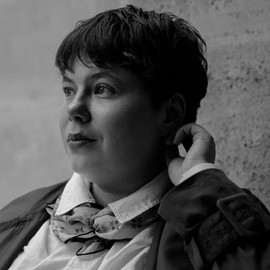##### Elena Dudukina
###### Consultant/Pharmacoepidemiologist

I am interested in women’s health, reproductive epidemiology, pharmacoepidemiology, causal inference, directed acyclic graphs, and R stats.

Previous